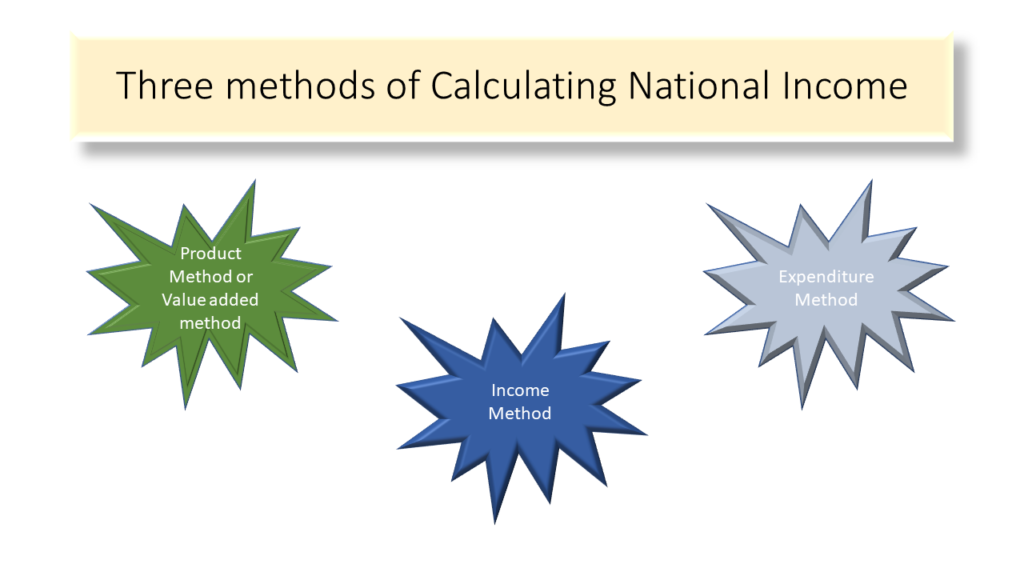# Methods for calculating National Income

There are 3 methods for measuring National Income

1. Production or Value added method
2. Income Method
3. Expenditure Method

GDP – Simply put , it is defined as the money value of goods and services produced in an economy. Suppose we want to find the GDP of India then we will add the money value of all the goods and services produced in India.

Value of Output – It means the money value of all the goods or services produced including raw materials

Intermediate consumption – The raw materials which are used in production and were produced by some one else in the supply chain. So here we have not done anything but we purchased that something and changed or modified the same into something else

Value Addition – (Value of Output – Intermediate consumption). This the production by our unit in economic sense. So out of the total product value only that much which is done by us will be considered as produced by us.

Example – Suppose I buy a cloth worth Rs 1000 and make a shirt from it worth Rs 1500. Here Value of Output is Rs 1500, Intermediate consumption is Rs 1000 and Value addition is Rs 500.

The value addition done by us is basically the cost of factors of production, i.e. Land, labour, capital and enterprise, hired by us. The entire Rs 500 gets distributed between the four factors of production.

This means that the total production/value addition done by us becomes equal to total income of factors of production.

Now if total income of factors of production is spent and there are no savings (assumed)  then it will mean that total income is also equal to total expenditure.

Combining all of the above we get triple identity

PRODUCTION/VALUE ADDITION = INCOME = EXPENDITURE

So we have three methods for measuring National Income